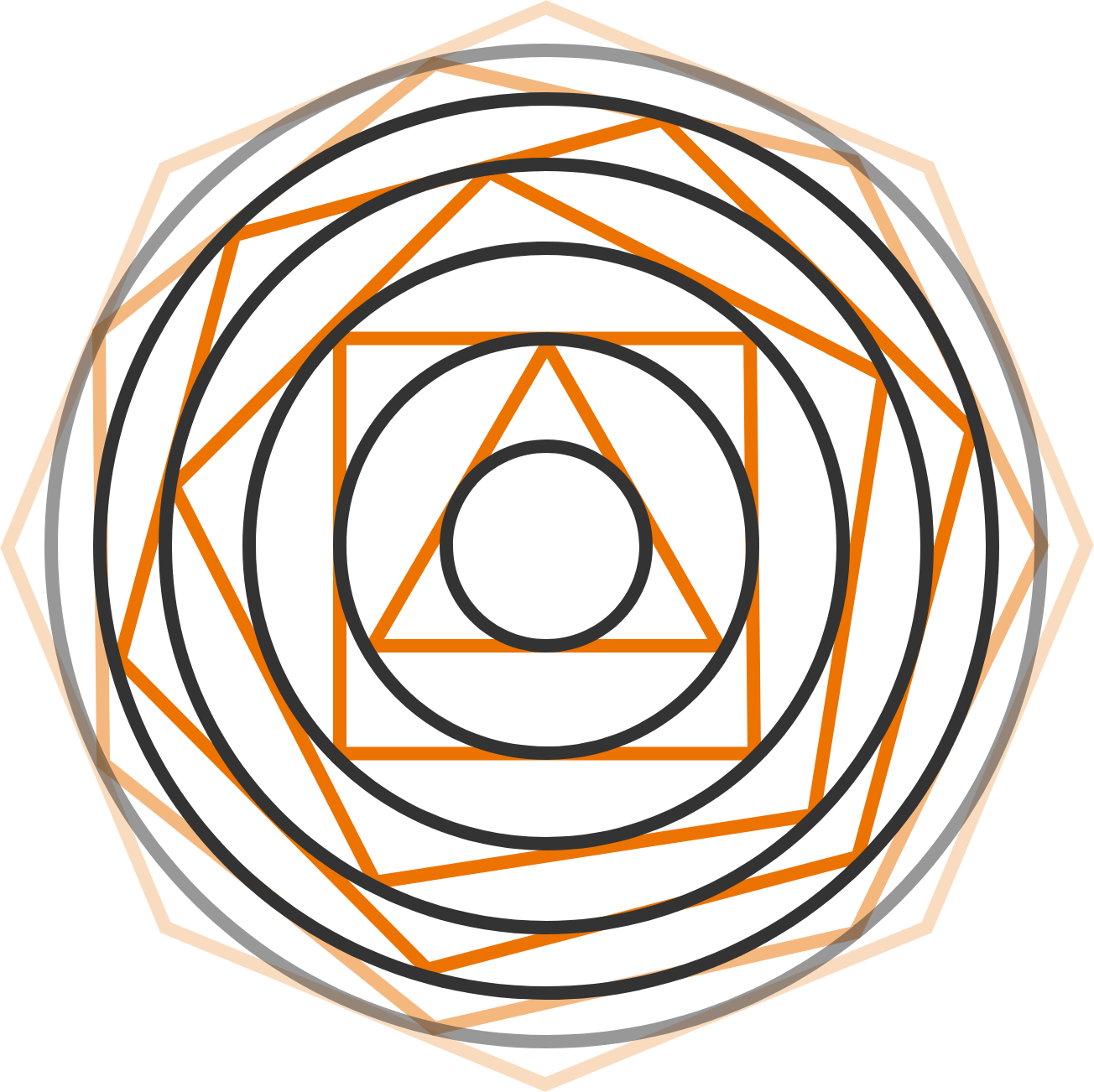# Nesting Shapes

Geometry Level 4I begin with a circle of radius $1 \text{ cm}$, which becomes the inscribed circle of an equilateral triangle, which I enclose with its circumcircle (the circle that touches all of its vertices). I enclose this circle with a square (so that each edge touches the circle once), and surround this with the square's circumcircle. I repeat this procedure with a pentagon, a hexagon, and so on forever (increasing the side number by one, until we reach an infinity-sided polygon).

What is the limiting area (in $\text{cm}^2$) of the "final" circle, rounded to a whole number?

×

Problem Loading...

Note Loading...

Set Loading...